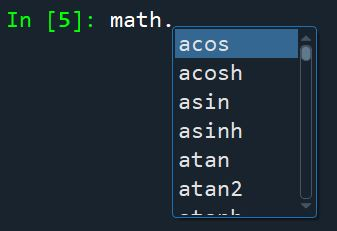## Python as a Calculator

Python contains functions found in any standard graphing calculator. An arithmetic operation is either addition, subtraction, multiplication, division, or powers between two numbers. An arithmetic operator is a symbol that Python reserves to mean one of the aforementioned arithmetic operations. These symbols are

• + for addition
• - for subtraction
• * for multiplication
• / for division
• ** for exponentiation.

Example

Let’s look at a few examples.

11+2 # addition

3

12**3 # exponentiation

8

13/4 # division

0.75


Note that the hash key # precedes a comment which will be ignored by the Python compiler.

### Order of Operations

An ‘order of operations’ is a standard order of precedence that different operations have in relationship to one another. Python also uses a similar type of rule known as PEMDAS 1. Powers are executed before multiplication and division, which are executed before addition and subtraction.

• P – Parentheses
• E – Exponentiation
• M – Multiplication
• D – Division
• S – Subtraction

The precedence of operators above is listed from High to low. For M and D, we perform (M)ultiplication or (D)ivision from left to right based on whichever operation comes first. Similarly, for A and S, we perform (A)ddition or (S)ubtraction from left to right based on whichever operation comes first.

Example

The following illustrates the fact that if M and D appear together without any parentheses, the order of operations will be from left to right.

142/7*2

12.0


However, it is always best to always use parentheses to precisely define the order you desire.

Example

Compute $$\frac{2 \times 3}{2^2 + \frac{6}{3} }$$

1(2*3)/(2**2 + 6/3)

1.0


## Mathematical Functions

Python has many basic mathematical functions like sin, cos, tan, asin, acos, atan, exp, log, log10 and sqrt stored in a module (explained in a later section) called math. To access these functions, we need to first import this module.

1import math


After importing the math module, we can now access the various math functions by typing math followed by a dot and the function desired.

Example

To compute $\sqrt{16} = 4$.

1math.sqrt(16)

4.0


Example

To compute $\cos{\pi} = -1.0$.

1math.cos(math.pi)

-1.0


Example

To compute $e ^{\ln(5)}$.

1math.exp(math.log(5))

4.999999999999999


Note that the result above is not 5.0 exactly due to Python’s inherent floating-point approximation. Also, the log function in Python refers to the natural logarithm. To compute the logarithm of a number ($a$) to any base, we use log(a,base).

Example

To compute $\log_2 8$.

1math.log(8,2)

3.0


Example

Division $\frac{17}{3}$.

117 / 3  # classic division returns a float

5.666666666666667


Example

Floor division.

117 // 3  # floor division discards the fractional part

5


Example

The following computes the remainder when 10 is divided by 3.

1math.remainder(10,3)

1.0


An alternative syntax is

110 % 3

1


where % is called the modulo symbol.

The complete list of Python’s math functions can be found here . Another way to see all available math functions is to type math followed by a dot and the tab key.1. PEDMAS is typically introduced in a course on precalculus. ↩︎